# JABBA: Just Another Bayesian Biomass Assessment

## Package Description

The details of the JABBA method are provided in https://www.sciencedirect.com/science/article/pii/S0165783618300845

The documentation for JABBA states: “The motivation for developing JABBA was to provide a user-friendly R to JAGS (Plummer) interface for fitting generalized Bayesian State-Space Surplus Production Models (SPMs) with the aim to generate reproducible stock status estimates and diagnostics. Building on recent advances in optimizing the fitting procedures through the development of Bayesian state-space modelling approaches, JABBA originates from a continuous development process of a Bayesian State-Space SPM tool that has been applied and tested in many assessments across oceans. JABBA was conceived in the Hawaiian Summer of 2015 as a collaboration between young researchers from South Africa and the Pacific Islands Fisheries Science Center (NOAA) in Honolulu, HI USA. The goal was to provide a bridge between age-structured and biomass dynamic models, which are still widely used. JABBA runs quickly and by default generates many useful plots and diagnostic tools for stock assessments.”

JABBA requires R as found on CRAN. R can be installed from https://cran.r-project.org/.

The recommended way to run R is using RStudio. After you have installed R, then install RStudio. It can be found at https://www.rstudio.com/products/rstudio/download/.

# Installation

A self-contained R package is available. There are relevant materials in the repository https://github.com/jabbamodel/JABBA.

A tutorial vignette is available from https://github.com/jabbamodel/JABBA/blob/master/Tutorial_Vignette.md.

Set up checking/installing packages functions

``````# Ordinary checking/installing function
Check_Install.packages <- function(pkg){
new.pkg <- pkg[!(pkg %in% installed.packages()[, "Package"])]
if (length(new.pkg))
install.packages(new.pkg,dependencies = TRUE) else print(paste0("'",pkg,"' has been installed already!"))
sapply(pkg, require, character.only = TRUE)
}

# github checking/installing function
Github_checkInstall.packages <- function(github_pkg){
pkg = tail(unlist(strsplit(github_pkg,"/")),1)
new.pkg <- pkg[!(pkg %in% installed.packages()[, "Package"])]
if (length(new.pkg))
devtools::install_github(github_pkg)
sapply(pkg, require, character.only = TRUE)
}``````

Installing packages from GitHub directly.

Check and install “devtools”

``Check_Install.packages("devtools")``
``##  "'devtools' has been installed already!"``
``## Loading required package: devtools``
``## Warning: package 'devtools' was built under R version 4.0.3``
``## Loading required package: usethis``
``````## devtools
##     TRUE``````

Check and install “JABBA” from github

``````# Check if existed and if not, install the required package TMBhelper:
github_pkg = "jabbamodel/JABBA"
Github_checkInstall.packages(github_pkg=github_pkg)``````
``## Loading required package: JABBA``
``````## JABBA
##  TRUE``````
``library(JABBA)``

On the GitHub site (which is the repository for this package on https://github.com/jabbamodel/JABBA), the package can be used to a) apply an integrated state-space tool for averaging multiple CPUE series (+SE); b) automatic fit multiple CPUE time series and associated standard errors; c) fit Fox, Schaefer or Pella-Tomlinson production functions (using input BMSY/K); d) implement Kobe-type biplot plotting functions; e) forecast under alternative TACs; f) report residual and MCMC diagnostics; g) estimate or fix the process variance; h) estimate additional observation variances for individual or grouped CPUE time-series; and i) implement time-block changes in selectivity.

The description below is based on fitting to our empirical catch and CPUE data. This is a simplified example of a single CPUE series and it mainly involves 1) automatically fitting one CPUE-time series given its associated standard errors; 2) assuming the Schaefer production function (one can also run with Fox or Pella-Tomlinson production functions); and 3) outputing the results of the model run in a local folder and ploting them.

Although we describe the steps below, there is a good description in https://github.com/jabbamodel/JABBA/blob/master/Tutorial%20Vignette.Rmd, which we recommend if you want to run the JABBA tutorial data following their instructions.

### Setup working directories and output folder labels

To be able to execute the core model, one needs to set up a working directory and output directory. The directory that contains this RMD file is set as the working directory. A sub-directory "_New" is created to host the model outputs.

In the next chunk we aspecify the model, such as what kind of plots to output.

``````# Set work directories as same as this file
File = "./"

# Set Assessment file: assessment folder within File that includes .csv input files
assessment = "OurTestData_New" ``````

Set up the models configurations.

Note: Our application involves settings with default values. If not given here, our test run will use the function’s default values.

``````#><>><>><>><>><>><>><>><>><>><>><>><>><>><>><>><>><>><>><>><>><>><>
# Graphic, Output, Saving (.RData) settings
#
#><>><>><>><>><>><>><>><>><>><>><>><>><>><>><>><>><>><>><>><>><>><>

KOBE.plot = TRUE # Produces JABBA Kobe plot
KOBE.type = c("ICCAT","IOTC") # ICCAT uses 3 colors; IOTC 4 (incl. orange)
Biplot= TRUE # Produces a "post-modern" biplot with buffer and target zones (Quinn & Collie 2005)
SP.plot = c("standard","phase") # Produces standard or 'Kobe phase' SP plot
save.trajectories =TRUE # saves posteriors of P=B/K, B/Bmsy and H/Hmsy as .RData object
harvest.label = c("Hmsy","Fmsy") # choose label preference H/Hmsy versus Fmsy
CPUE.plot= TRUE # Runs state-tool to produce "alligned" multi-CPUE plot
meanCPUE = FALSE # Uses averaged CPUE from state-space tool instead of individual indices
Projection = TRUE # Use Projections: requires to define TACs vectors
save.projections = TRUE # saves projection posteriors as .RData object
catch.metric = "(t)" # Define catch input metric e.g. (tons) "000 t" etc
Reproduce.seed = TRUE # If FALSE a random seed assigned to each run, if TRUE set.seed(123)
# P_bound = c(0.02,1)  # Soft penalty bounds for P
# Save entire posterior as .RData object
save.all = FALSE # (if TRUE, a very large R object of entire posterior is saved)
#><>><>><>><>><>><>><>><>><>><>><>><>><>><>><>><>><>><>><>><>><>><>``````

### Surplus Production model specification

Three surplus production models can be chosen from: Schaefer, Fox, and Pella-Tomlinsson. To allow for depensarion,set Plim = Blim/K, the depletion where recruitment may become impaired (e.g. Plim = 0.25). Choose Plim = 0 to implement the conventional Schaefer, Fox, Pella-Tomlinson models.

The following are required to read in the data. In this case,

``````#--------------------------------------------------
#--------------------------------------------------

# Use SEs from csv file for abudance indices (TRUE/FALSE)
SE.I = FALSE

# Remove fleet from catch
catch = catch[,-c(2)]
names(catch) <-"Catch"

# Remove lines where catch is 0
catch = catch[catch\$Catch>0,]

# Remove fleet/CV from cpue
cpue = cpue[,-c(2,4)]
names(cpue) = "CPUE"

# need to align the cpue data length to catch length

cpue = merge(catch,cpue,all=T)
cpue=cpue[,-2]``````

### Set up priors

We use similar settings to authors regarding the priors for unfished biomass K, initial depletion (I.e. P1= B/B0), q, r and observation error, except set the K prior smaller as 20,000.

We set projection to FALSE. However, the TAC-relevant initial values have to be retained to keep the model running.

Note: If not given here, our test run will use the function’s default values.

``````#------------------------------------------------
# Prior for unfished biomass K
#------------------------------------------------
# The option are:
# a) Specify as a lognormal prior with mean and CV
# b) Specify as range to be converted into lognormal prior

K.dist = c("lnorm","range")   # using lnorm

# if lnorm use mean and CV; if range use lower,upper bound
#K.prior = c(200000,1)
K.prior = c(20000,1)

#-----------------------------------------------------------
# mean and CV and sd for Initial depletion level P1= SB/SB0
#-----------------------------------------------------------
# Set the initial depletion prior B1/K
# To be converted into a lognormal prior (with upper bound at 1.1)

psi.dist= c("lnorm","beta")
# specify as mean and CV
psi.prior = c(1,0.25)

#--------------------------------------------------------------
# Determine estimation for catchability q and observation error
#--------------------------------------------------------------
# Assign q to CPUE
sets.q = 1:(ncol(cpue)-1)

#----------------------------------------------------
# Determine r prior
#----------------------------------------------------
# The option are:
# a) Specifying a lognormal prior
# b) Specifying a resiliance category after Froese et al. (2017; CMSY)
# Resilience: "Very low", "Low", "Medium", High" (requires r.range = TRUE)

# use  lognormal(mean,stdev) or  range (min,max) or
r.dist = c("lnorm","range")

r.prior = c(0.42,0.37)

#><>><>><>><>><>><>><>><>><>><>><>><>><>><>><>><>><>><>><>><>>
# Observation Error
#><>><>><>><>><>><>><>><>><>><>><>><>><>><>><>><>><>><>><>><>>

sigma.est = TRUE

# Series
sets.var = 1:(ncol(cpue)-1) # estimate individual additional variance

# As option for data-weighing
# minimum fixed observation error for each variance set (optional choose 1 value for both)
fixed.obsE = c(0.2) # Important if SE.I is not available

# Total observation error: TOE = sqrt(SE^2+sigma.est^2+fixed.obsE^2)

#><>><>><>><>><>><>><>><>><>><>><>><>><>><>><>><>><>><>><>><>>
# Process Error
#><>><>><>><>><>><>><>><>><>><>><>><>><>><>><>><>><>><>><>><>>
#Estimate set sigma.proc == True
sigma.proc = TRUE
# Determines if process error deviation are estimated for all years (TRUE)
# or only from the point the first abundance index becomes available (FALSE)
proc.dev.all = FALSE
#------------------------------------------
if(sigma.proc == TRUE){
igamma = c(4,0.01) #specify inv-gamma parameters

# Process error check
gamma.check = 1/rgamma(1000,igamma,igamma)
# check mean process error + CV
mu.proc = sqrt(mean(gamma.check)); CV.proc = sd(sqrt(gamma.check))/mean(sqrt(gamma.check))

# check CV
round(c(mu.proc,CV.proc),3)
quantile(sqrt(gamma.check),c(0.1,0.9))
}else{
sigma.proc = 0.07 #IF Fixed: typically 0.05-0.15 (see Ono et al. 2012)
}
#--------------------------------------------
#><>><>><>><>><>><>><>><>><>><>><>><>><>><>><>><>><>><>><>><>>
# Optional: Do TAC Projections
#><>><>><>><>><>><>><>><>><>><>><>><>><>><>><>><>><>><>><>><>>
#Projection = TRUE # Switch on by Projection = TRUE

Projection = TRUE # Switch on by Projection = TRUE

# Check final year catch
catch[nrow(catch),]

# Set range for alternative TAC projections
TACs = seq(1000,2000,200) #example

# Intermittent TAC to get to current year
#TACint = mean(catch[nrow(catch)-3,2]:catch[nrow(catch),2]) # avg last 3 years
TACint = 1493 # Catch for 2000
# Set year of first TAC implementation
imp.yr = 2002
# Set number of projections years
pyrs = 10``````

### Execute model and produce output

We use the same MCMC settings as the author’s example, implement three models (“Schaefer”,“Fox”,“Pella”) and store the outputs in the local folders. The “Fox” model results are plotted as follows.

Note: If not given here, our test run will use the function’s default values.

Run “Schaefer” model

`````` output.dir = file.path(File,assessment,"Schaefer")
dir.create(output.dir,showWarnings = FALSE)

# build_jabba function default values:

# function (catch = NULL, cpue = NULL, se = NULL, assessment = "bet_example",
#           scenario = "test", model.type = c("Schaefer", "Fox", "Pella", "Pella_m"),
#           catch.cv = 0.1, Plim = 0, r.dist = c("lnorm", "range"),
#           r.prior = c(0.2, 0.5), K.dist = c("lnorm", "range"),
#           K.prior = NULL, psi.dist = c("lnorm", "beta"),
#           psi.prior = c(0.9, 0.25), b.prior = c(FALSE, 0.3, NA,
#               c("bk","bbmsy", "ffmsy")), BmsyK = 0.4, shape.CV = 0.3,
#           sets.q = 1:(ncol(cpue) - 1), sigma.est = TRUE,
#           sets.var = 1:(ncol(cpue) -1),
#           fixed.obsE = ifelse(is.null(se), 0.1, 0.001), sigma.proc = TRUE,
#           proc.dev.all = TRUE, igamma = c(4, 0.01), projection = FALSE,
#           TACs = NULL, TACint = NULL, imp.yr = NULL, pyrs = NULL,
#           P_bound = c(0.02,1.3), sigmaobs_bound = 1, sigmaproc_bound = 0.2,
#           q_bounds = c(10^-30,

jbinput = build_jabba(catch=catch,cpue=cpue,
assessment=assessment,scenario = Scenario,
model.type = "Schaefer",sigma.est = TRUE,
K.prior=K.prior, psi.prior = psi.prior,r.prior = r.prior,
proc.dev.all=proc.dev.all,projection=Projection,
TACs=TACs,TACint = TACint,imp.yr=imp.yr,pyrs=pyrs,
fixed.obsE = 0.2,igamma = igamma)``````
``````##
##  ><> Prepare JABBA input data <><
##
##
##  ><> Assume Catch with error CV =  0.1  <><
##
##
##  ><> SE.I=FALSE: Creatinng SE dummy matrix <><
##
##
##  ><> Model type: Schaefer  <><
##
##  ><> Shape m = 2
##
##  ><> K prior mean = 20000 and CV = 1 (log.sd =  0.8325546 )
##
##  ><> r prior mean = 0.42 and CV = 0.3830319 (log.sd =  0.37 )
##
##  ><> Psi (B1/K) prior mean = 1 and CV = 0.25 with lnorm destribution
##
##
##
##  ><> ALWAYS ENSURE to adjust default settings to your specific stock <><
## ``````
``````# Fit JABBA (here mostly default value - careful)

# fit_jabba function default values:
# function (jbinput, ni = 30000, nt = 5, nb = 5000, nc = 2, init.values = FALSE,
#          init.K = NULL, init.r = NULL, init.q = NULL, peels = NULL,
#          save.all = FALSE, save.jabba = FALSE, save.csvs = FALSE,
#          save.prjkobe = FALSE, output.dir = getwd(), quickmcmc = FALSE)

codoid_Schaefer = fit_jabba(jbinput,save.jabba=TRUE,output.dir=output.dir,
quickmcmc = TRUE) # quick run``````
``````## Compiling model graph
##    Resolving undeclared variables
##    Allocating nodes
## Graph information:
##    Observed stochastic nodes: 96
##    Unobserved stochastic nodes: 159
##    Total graph size: 2863
##
## Initializing model
##
##
## ><> Produce results output of Schaefer model for OurTestData_New Scenario1 <><
##
##  ><> compiling Future Projections under fixed quota <><
##
##
## ><> Scenario Scenario1_Schaefer completed in 0 min and 30 sec <><``````
``````# Plot all to local folder
jabba_plots(jabba=codoid_Schaefer,output.dir = output.dir)``````
``````##
## ><> jbplot_catch()  <><``````
``````##
## ><> jbplot_catcherror()  <><``````
``````##
## ><> jbplot_cpue() - fits to CPUE <><``````
``````##
## ><> jbplot_logfits()  <><``````
``````##
## ><> jbplot_mcmc() - mcmc chains  <><``````
``````##
## ><> jbplot_ppist() - prior and posterior distributions  <><``````
``````##
## ><> jbplot_procdev() - Process error diviations on log(biomass)  <><``````
``````##
## ><> jbplot_trj() - B trajectory  <><``````
``````##
## ><> jbplot_trj() - F trajectory  <><``````
``````##
## ><> jbplot_trj() - BBmsy trajectory  <><``````
``````##
## ><> jbplot_trj() - FFmsy trajectory  <><``````
``````##
## ><> jbplot_trj() - BB0 trajectory  <><``````
``````##
## ><> jbplot_spphase() - JABBA Surplus Production Phase Plot  <><``````
``````##
## ><> jbplot_residuals() - JABBA residual plot  <><``````
``````##
## ><> jbplot_staresiduals() - standardized residuals  <><``````
``````##
## ><> jbplot_runstest()   <><``````
``````##
## ><> jbplot_kobe() - Stock Status Plot  <><``````
``````## png
##   2``````

## Results for Schaefer model

### Plotting catch

``````# Make individual plots
jbplot_catch(codoid_Schaefer)``````
``````##
## ><> jbplot_catch()  <><``````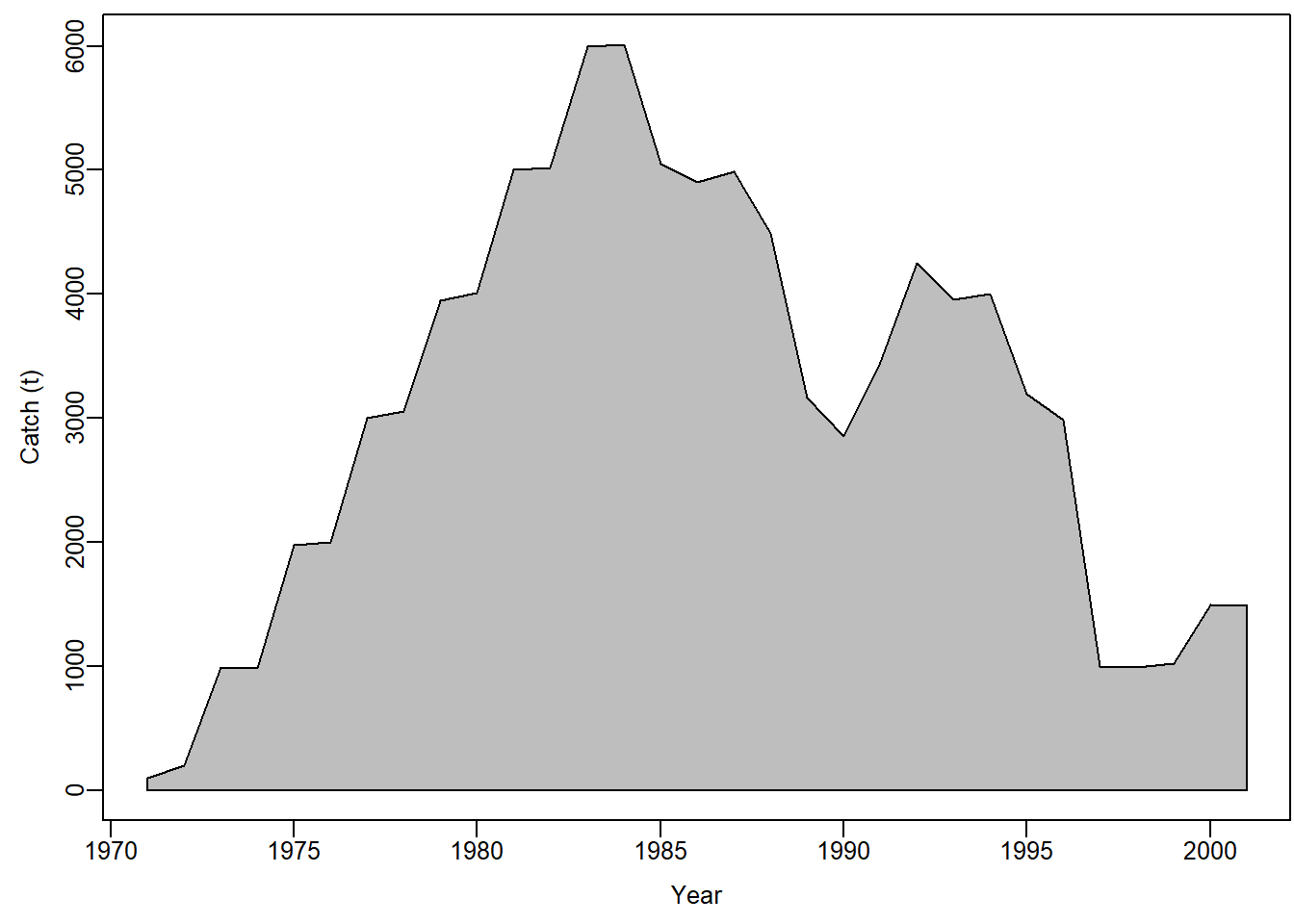### Plotting State-Space CPUE fits

``jbplot_cpuefits(codoid_Schaefer)``
``````##
## ><> jbplot_cpue() - fits to CPUE <><``````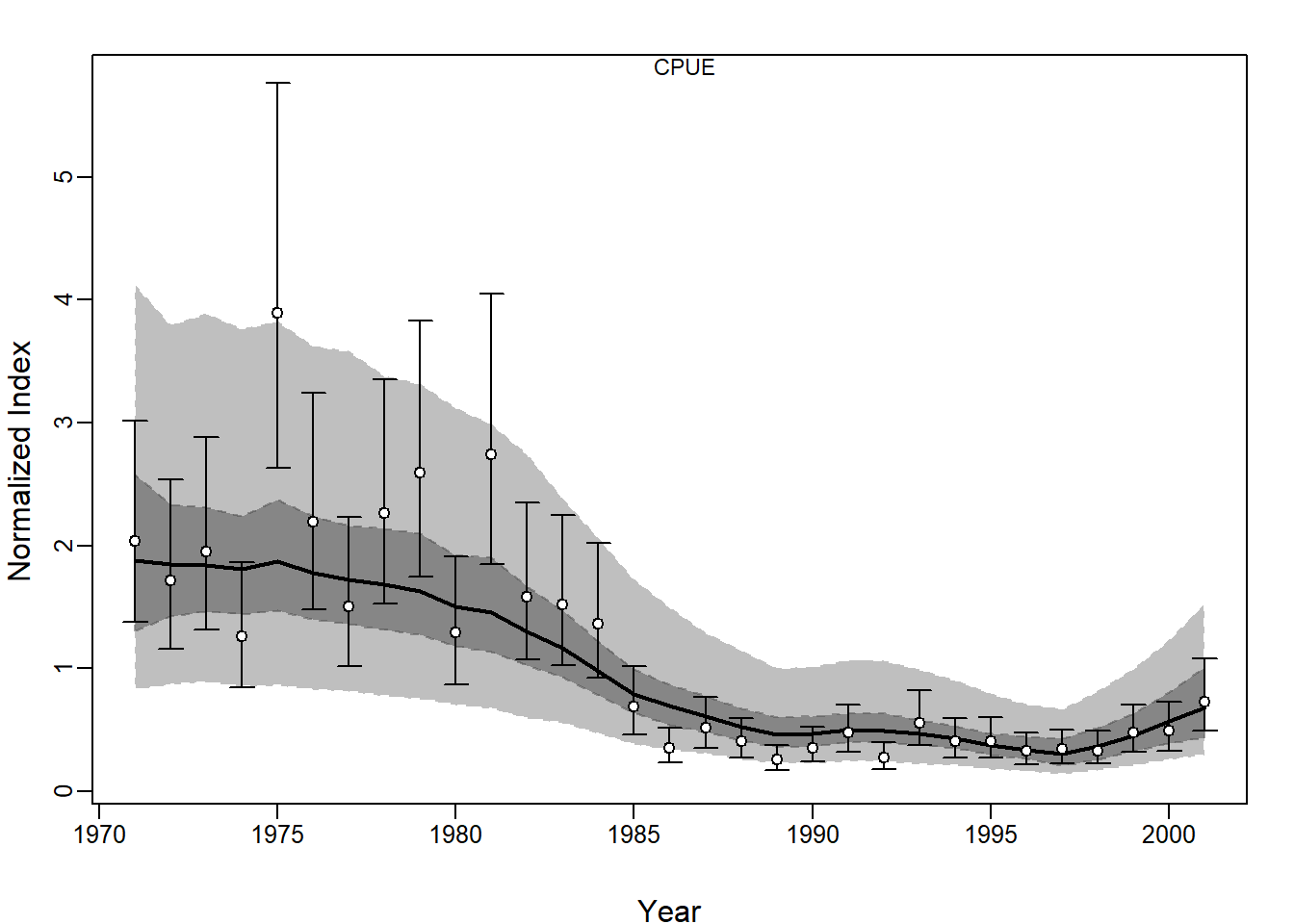### Plot posteriors

``jbplot_ppdist(codoid_Schaefer)``
``````##
## ><> jbplot_ppist() - prior and posterior distributions  <><``````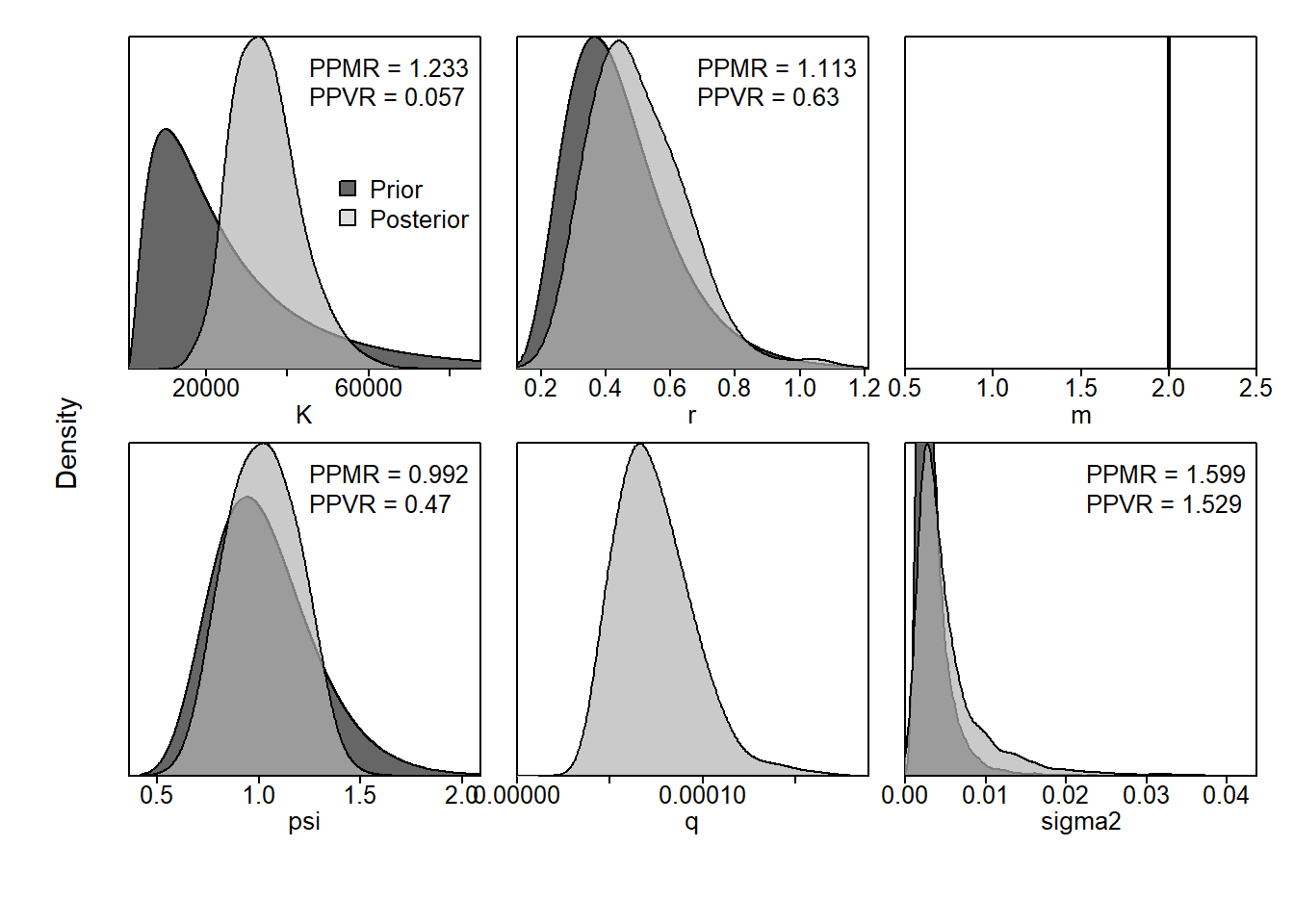### plot MCMC chains of posteriors

``jbplot_mcmc(codoid_Schaefer)``
``````##
## ><> jbplot_mcmc() - mcmc chains  <><``````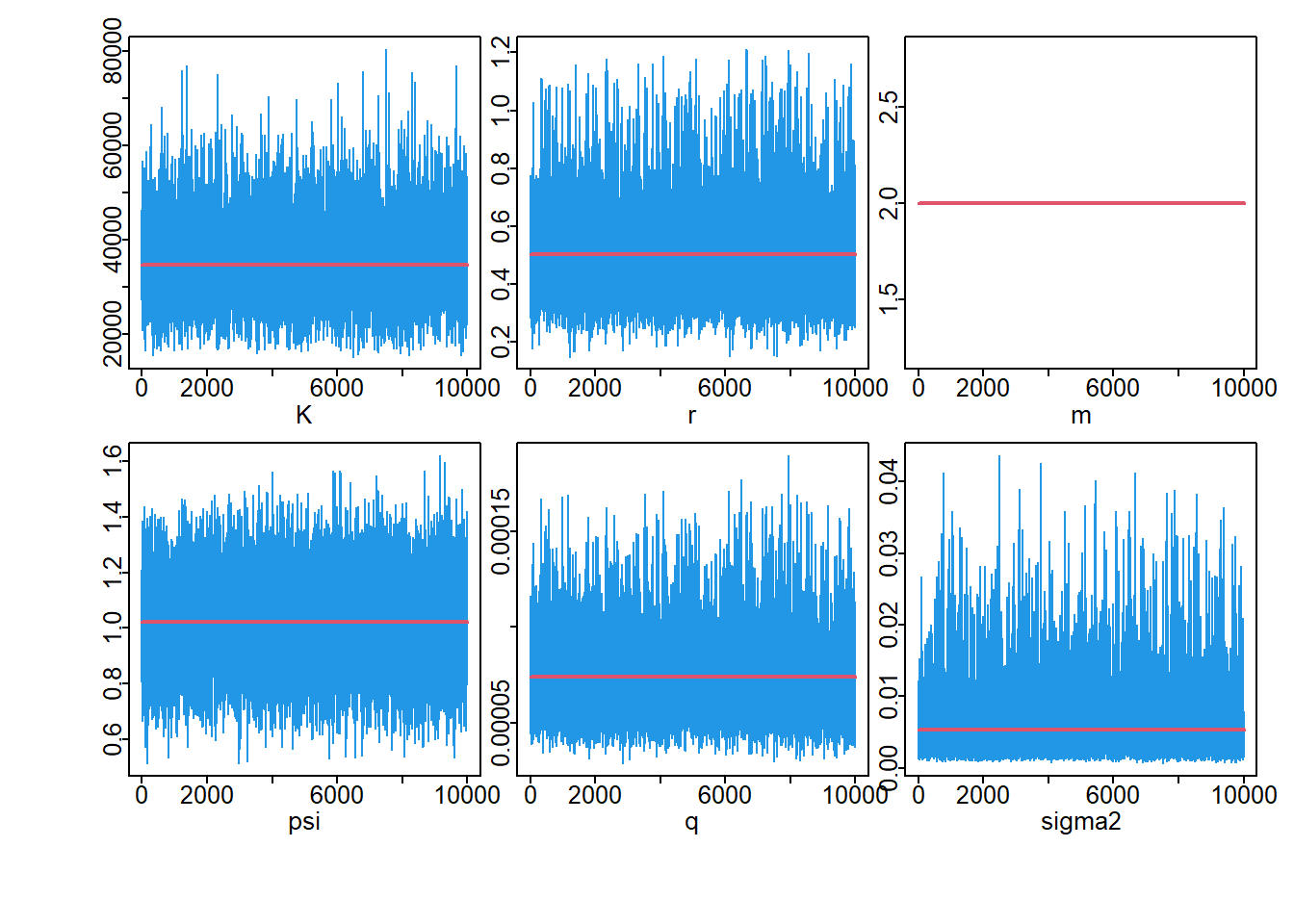### Log CPUE fitting

``jbplot_logfits(codoid_Schaefer)``
``````##
## ><> jbplot_logfits()  <><``````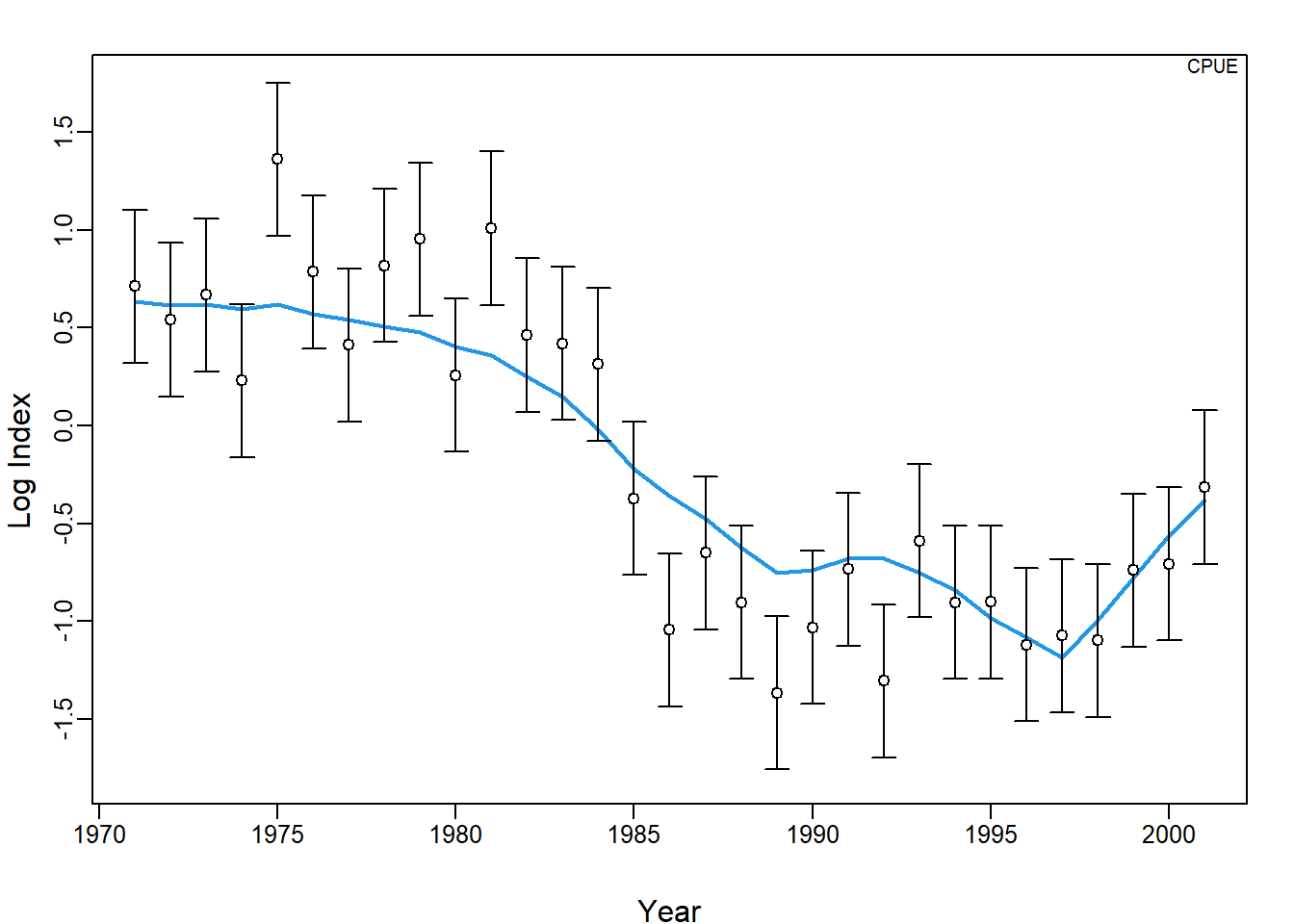### JABBA-residual plot

``jbplot_residuals(codoid_Schaefer)``
``````##
## ><> jbplot_residuals() - JABBA residual plot  <><``````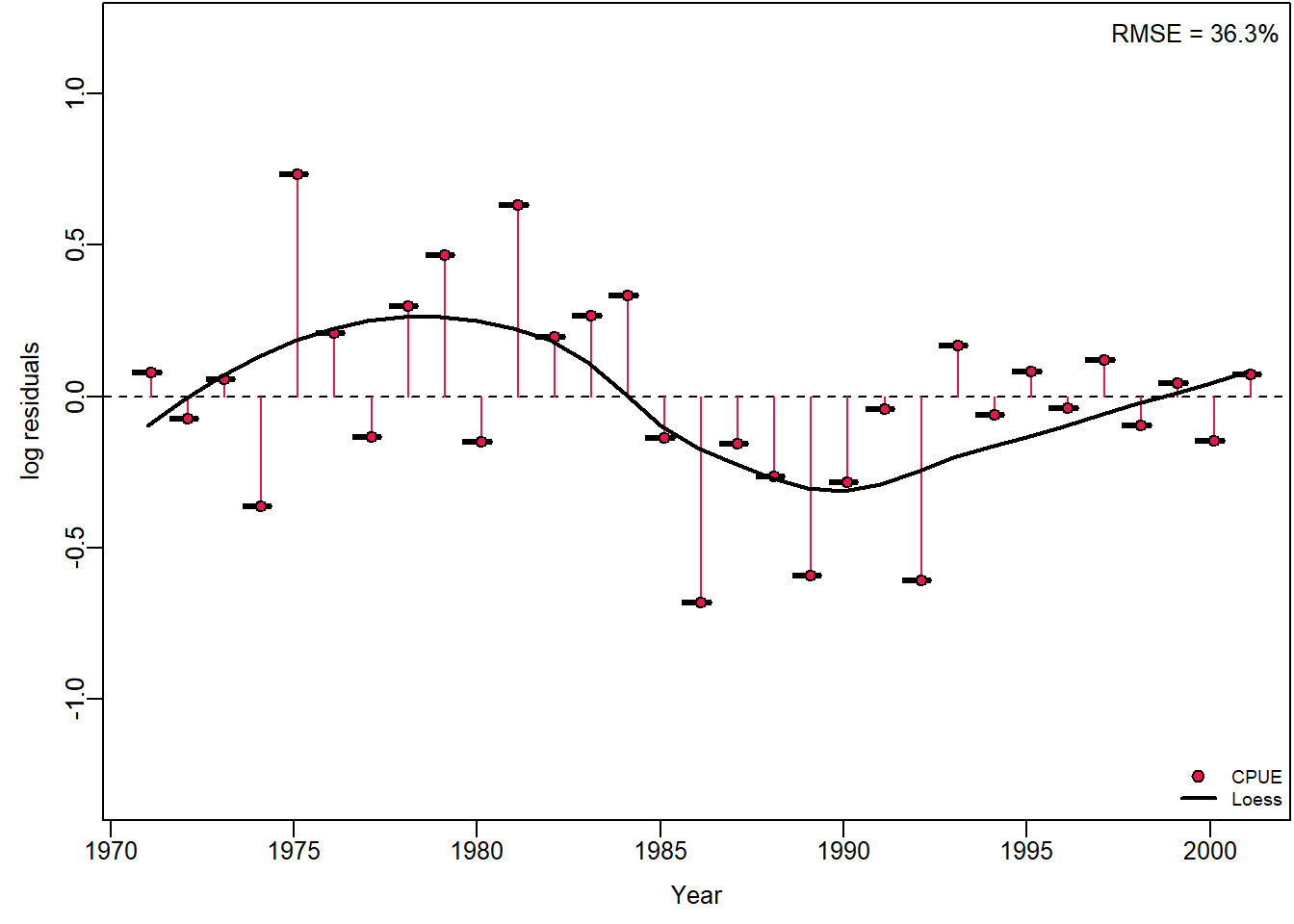``jbplot_stdresiduals(codoid_Schaefer)``
``````##
## ><> jbplot_staresiduals() - standardized residuals  <><``````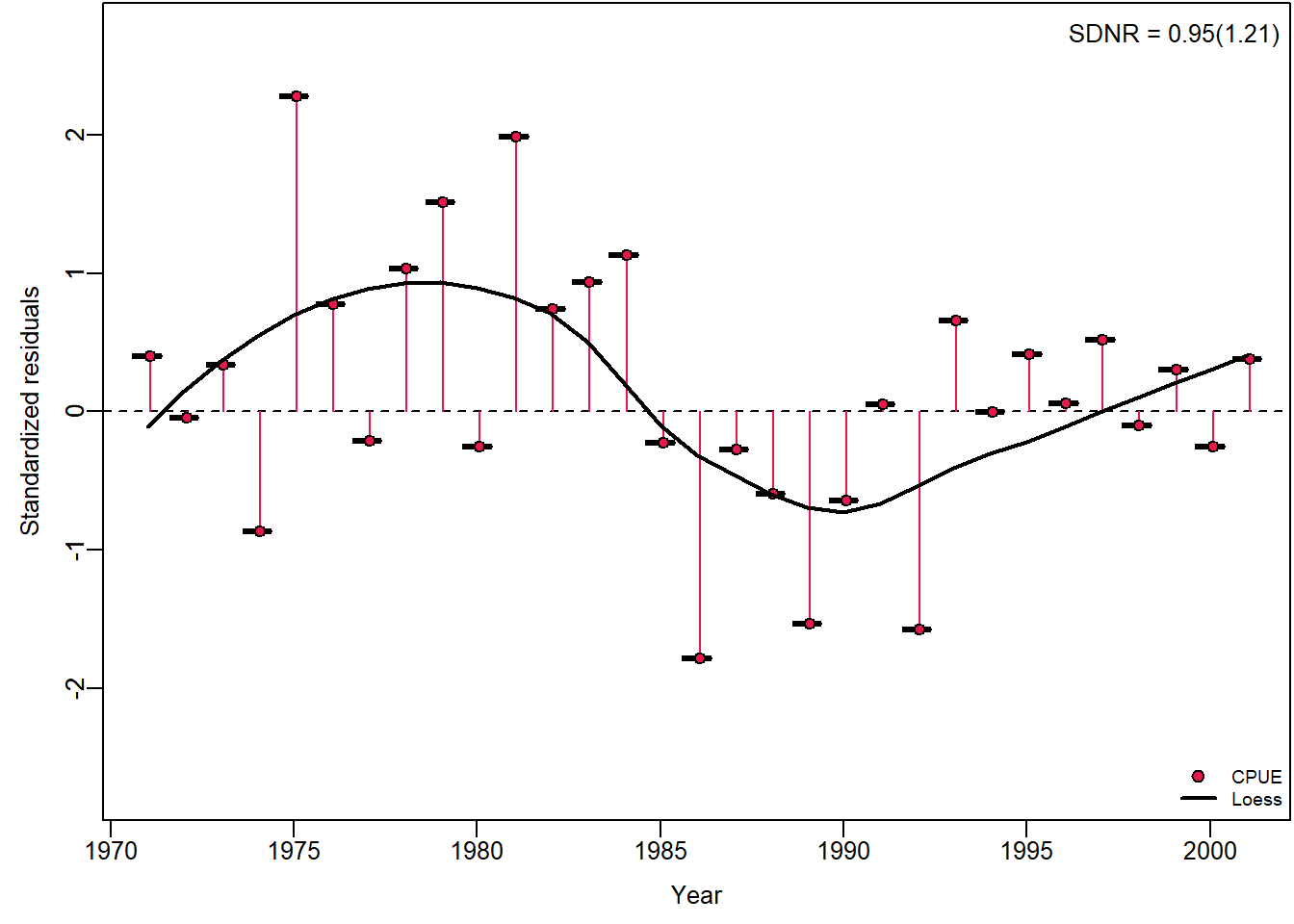### Plot process error deviation

``jbplot_procdev(codoid_Schaefer)``
``````##
## ><> jbplot_procdev() - Process error diviations on log(biomass)  <><``````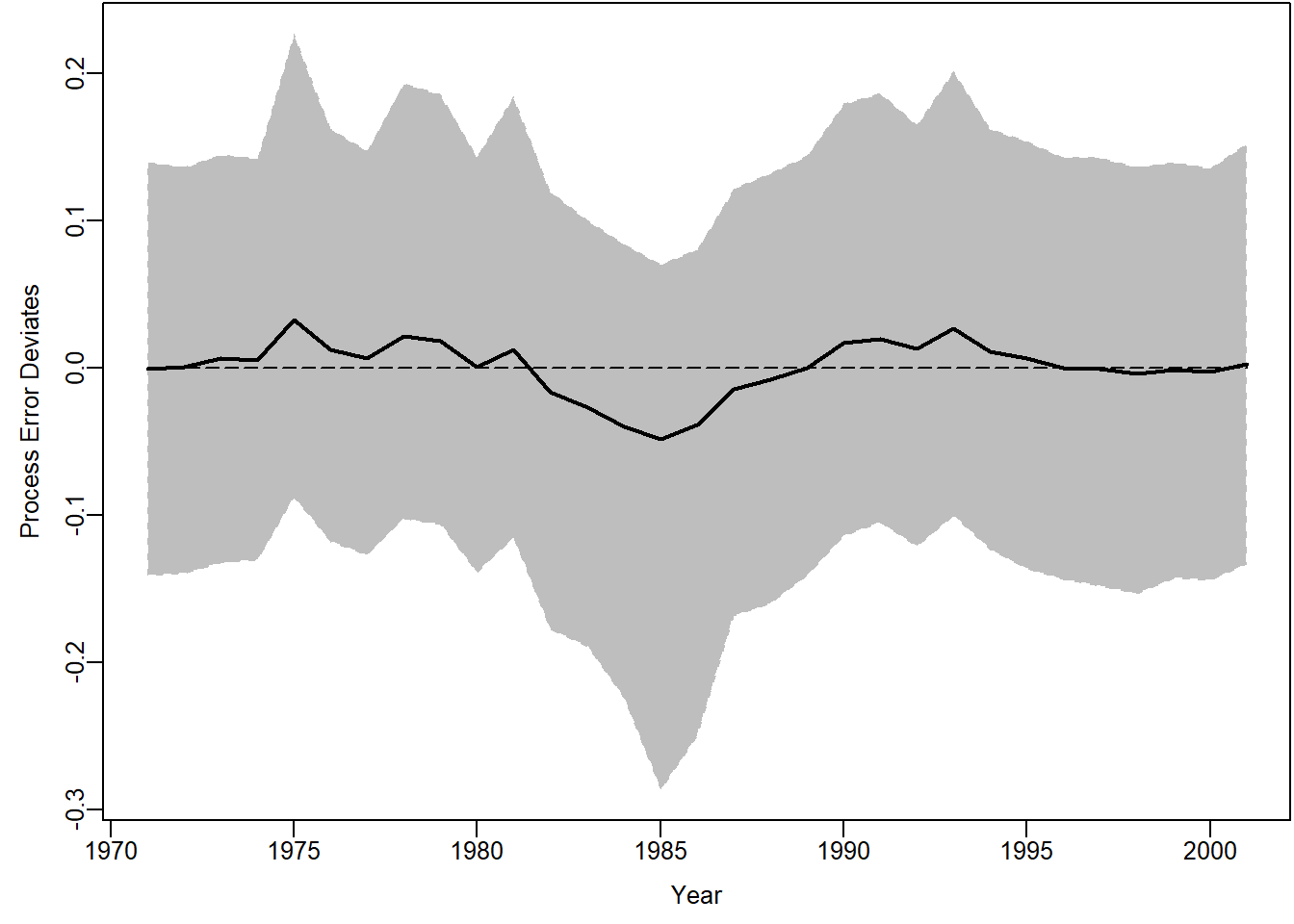### Plot Biomass time series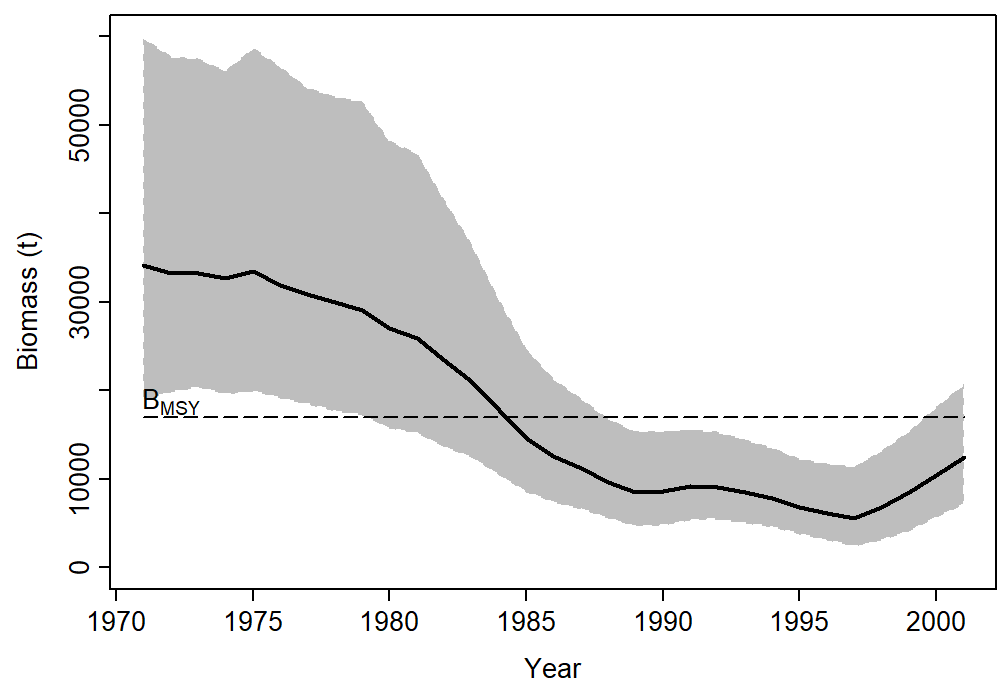### Plot B/BMSY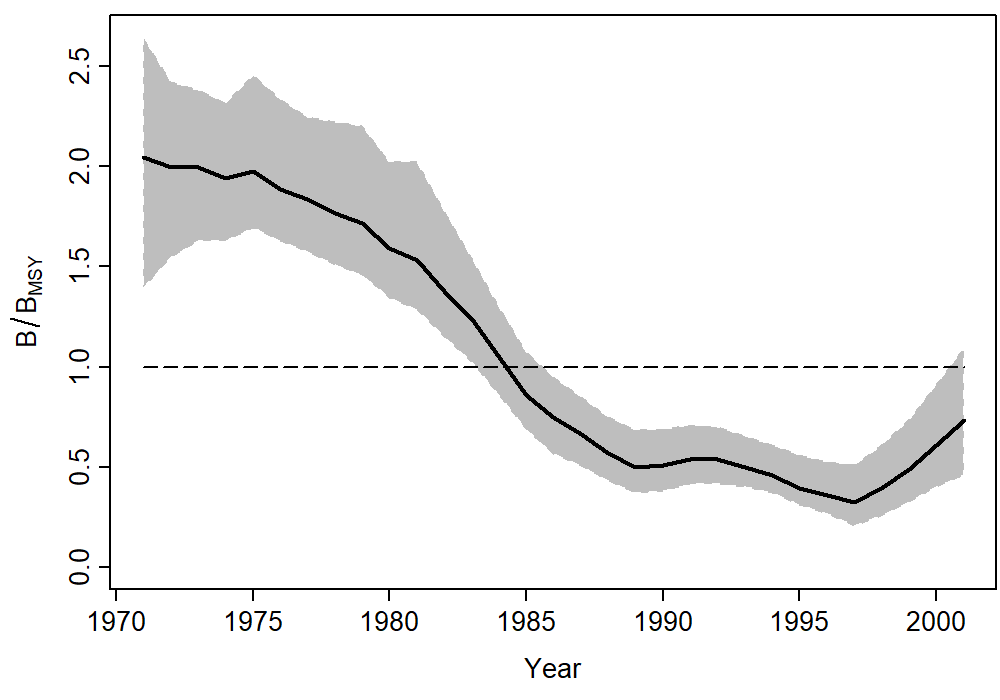### Plot F/FMSY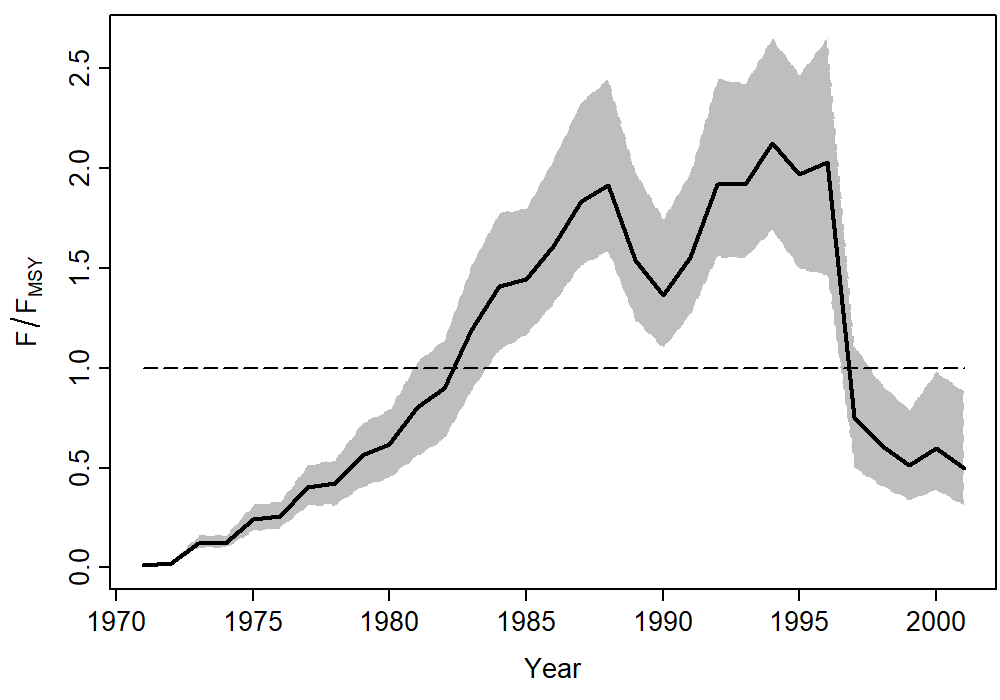### Plot projection of Biomass status given the TAC

``jbplot_prj(codoid_Schaefer,type = c("BB0"))``
``````##
## ><> jbplot_prj() - BB0 trajectory  <><``````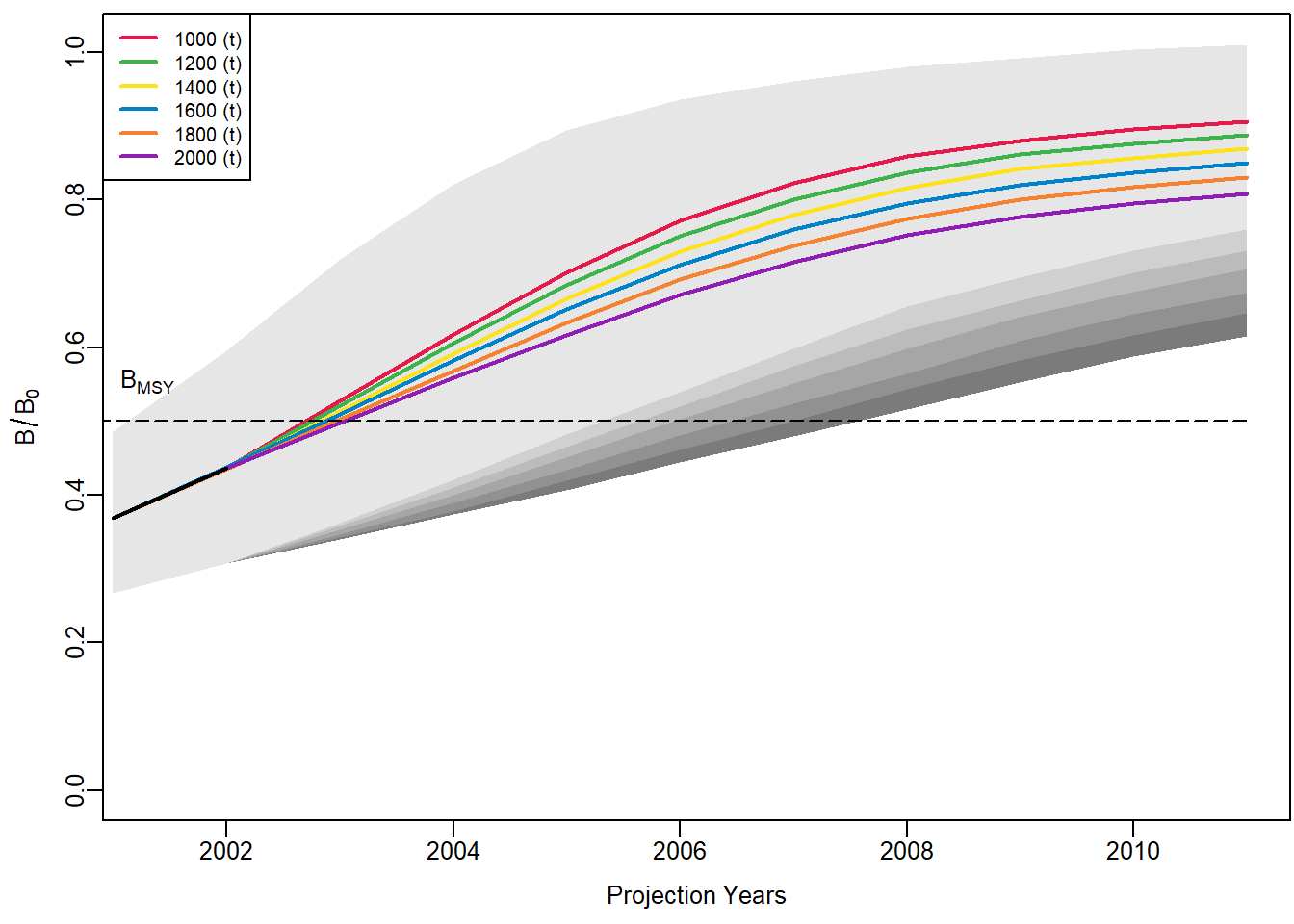``jbplot_prj(codoid_Schaefer,type = c("BBmsy"))  ``
``````##
## ><> jbplot_prj() - BBmsy trajectory  <><``````